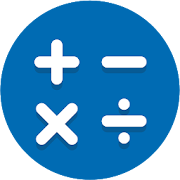# NT Calculator

## NT Calculator

Author
Thanh Le Tran Ngoc
Requirement
Android +
In-App Purchases
No
Rating
4.5
50,000
Highest Price
\$2.49
Lowest Price
\$0.00

\$2.49

Category:

## Product Description

Dear you,

This Extensive Calculator app allows you to easily handle all the calculations necessary for everyday life with a single application. A Calculator app with a simple interface and practical functions! It can replace your default calculator.

This calculator app doesn’t require ANY PERMISSION on your phone. And it is light and fast.

With Extensive Calculator Pro on your Android, you do not need to bring your calculator anymore. This calculator app serves you everything from A – Z.

List of calculators currently supported:
1. Standard Calculator
• Quick and easy.
• Basic features such as: %, +, -, x, /, 0 – 9, ( and ).

2. Scientific Calculator
• All features such as: sin, cos, tan, %, !, 0-9, (, ), RAD and DEG, log, ln, Mod, +, -, x, /, SQRT, SQR, signed,…
• You can use EXP is E (sam as 10 ^ ) to calculate the large decimal number. If you need to get the previous result of the calculator, you can use the ANS button.

• Covert from one thing to the other things is very simple.
• Base 2, 8 ,10, 16

4. Time Calculator
• Three primary functions is the hour (h), minute (m), second (s).
• History is available. And you can reuse by pressing calculation history (this feature is available in standard, scientific and time calculator).

5. Loan Calculator
• Suitable for car, bank loan
• Three primary input are loan amount, interest rate, loan term.

6. Date Calculator
• Calculate the difference between two dates.

7. Unit Converter
Supports many Unit of measurement commonly used in everyday life such as:
• Weight converter: kg to st, lbs to grams, lbs to kilograms, …
• Length converter: size converter, meters to kilometers, …
• Speed converter: km/h, cm/s, m/s, m/s to km/h, …
• Temperature converter: c to f, f to c, c to Kelvin, …
• Angle converter: the degree to radian, convert radians to degrees, …
• Data converter: byte to bit, bit to byte, …
• Area converter: km2 to m2, 1 hectare to km2, cm2 to m2, …
• Time converter: minute to second, year to second, …
• Energy converter: joule, kwh, …
• Pressure converter: atmospheres, bars, kilopascals, pascals, …
• Volume converter: gallons to qts, teaspoon, cups, liters, cubic feet, …
• Fuel Converter: many units,…